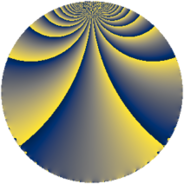# Properties

 Label 120.2.bLevel $120$ Weight $2$ Character orbit 120.b Rep. character $\chi_{120}(11,\cdot)$ Character field $\Q$ Dimension $16$ Newform subspaces $2$ Sturm bound $48$ Trace bound $2$

# Related objects

## Defining parameters

 Level: $$N$$ $$=$$ $$120 = 2^{3} \cdot 3 \cdot 5$$ Weight: $$k$$ $$=$$ $$2$$ Character orbit: $$[\chi]$$ $$=$$ 120.b (of order $$2$$ and degree $$1$$) Character conductor: $$\operatorname{cond}(\chi)$$ $$=$$ $$24$$ Character field: $$\Q$$ Newform subspaces: $$2$$ Sturm bound: $$48$$ Trace bound: $$2$$ Distinguishing $$T_p$$: $$23$$

## Dimensions

The following table gives the dimensions of various subspaces of $$M_{2}(120, [\chi])$$.

Total New Old
Modular forms 28 16 12
Cusp forms 20 16 4
Eisenstein series 8 0 8

## Trace form

 $$16q + 2q^{4} - 6q^{6} + O(q^{10})$$ $$16q + 2q^{4} - 6q^{6} - 2q^{10} - 16q^{12} - 14q^{16} + 4q^{18} - 8q^{19} + 28q^{22} - 2q^{24} + 16q^{25} - 24q^{27} - 4q^{28} - 8q^{30} - 8q^{33} - 6q^{36} - 14q^{40} + 32q^{42} - 16q^{46} - 4q^{48} - 32q^{49} + 40q^{51} + 40q^{52} + 26q^{54} - 8q^{57} - 4q^{58} - 14q^{60} + 50q^{64} - 16q^{66} - 64q^{67} + 12q^{70} + 32q^{72} - 16q^{73} - 24q^{76} + 24q^{78} + 16q^{81} - 8q^{82} + 56q^{84} + 28q^{88} + 18q^{90} + 48q^{91} - 80q^{94} - 26q^{96} + 16q^{97} + 32q^{99} + O(q^{100})$$

## Decomposition of $$S_{2}^{\mathrm{new}}(120, [\chi])$$ into newform subspaces

Label Dim. $$A$$ Field CM Traces $q$-expansion
$$a_2$$ $$a_3$$ $$a_5$$ $$a_7$$
120.2.b.a $$8$$ $$0.958$$ 8.0.1649659456.5 None $$-1$$ $$0$$ $$8$$ $$0$$ $$q-\beta _{1}q^{2}-\beta _{6}q^{3}+(\beta _{1}+\beta _{3}+\beta _{4})q^{4}+\cdots$$
120.2.b.b $$8$$ $$0.958$$ 8.0.1649659456.5 None $$1$$ $$0$$ $$-8$$ $$0$$ $$q+\beta _{1}q^{2}-\beta _{4}q^{3}+(\beta _{1}+\beta _{3}+\beta _{4})q^{4}+\cdots$$

## Decomposition of $$S_{2}^{\mathrm{old}}(120, [\chi])$$ into lower level spaces

$$S_{2}^{\mathrm{old}}(120, [\chi]) \cong$$ $$S_{2}^{\mathrm{new}}(24, [\chi])$$$$^{\oplus 2}$$